# Bihar Board Class 9 Science Solutions Chapter 10 Gravitation

## BSEB Bihar Board Class 9 Science Solutions Chapter 10 Gravitation

Bihar Board Class 9 Science Solutions Chapter 10 Gravitation Textbook Questions and Answers, Additional Important Questions, Notes.

### Bihar Board Class 9 Science Chapter 10 Gravitation InText Questions and Answers

Page 134

Question 1.
State the universal law of gravitation.
Every object in the universe attracts every other object in the universe with a force which is directly proportional to the product of their masses and inversely proportional to t he square of the distance between them. The force is along the line joining the centres of two objects.

Question 2.
Write the formula to find the magnitude of the gravitational force between the earth and an object on the surface of the earth.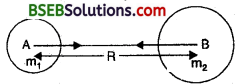Consider two bodies A andBof masses m1 and m2 separated by a distance R, then
F ∝ m1 x m2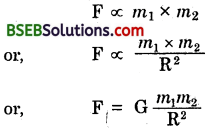where, G is called the universal gravitational constant.

Page 136

Question 1.
What do you mean by free fall?
Whenever objects fall towards the earth, we say that the objects are in free fall.

Question 2.
What do you mean by acceleration due to gravity?
The acceleration acquired by a body falling freely towards the earth is called acceleration due to gravity: It is denoted by ‘g’ The acceleration due to gravity is described as,
Acceleration due to gravity
= g = $$\frac{\mathrm{GM}_{e}}{\mathrm{R}^{2}}$$
where Me = Mass of earth
Thus, ‘g’ does not depend uport the mass of the falling object, i.e., the acceleration due to gravity is same for all the freely falling bodies.Page 138

Question 1.
What are the differences between the mass of an object and its weight.
Mass :

• Mass is the amount of matter contained in a body.
•  Mass in any object remains constant at all places.
• It is measured in kilogram (kg) unit.
• Mass is measured with the help of a physical s balance.

Weight :

• Weight is the force with which a body is attracted towards the earth.
• Weight of an object varies from place to place.
• It is measured in newton (N) unit.
• Weight is measured by using a spring balance.

Question 2.
Why is the weight of an object on the moon $$\frac {1}{6}$$ th its weight on the earth ?
By definition,
W = m x g
where W = Weight of object
m = Mass of an object .
g = Gravitational force
The mass of an object is constant and does not change on the earth or on the moon. Hence, the weight of the object depends on the gravitational force. As the gravitational force of the moon is about one sixth of what it is on the earth, the weight of the object on the moon is also $$\frac {1}{6}$$ th the weight on the earth.page 141

Question 1.
Why is it difficult to hold a school bag having a strap made of a thin and strong string ?
A school bag has wide strap so that weight of the bag may fall over a large area of the shoulder of the child producing less pressure on the shoulder. As a result it will be comfortable to carry the bag.

Question 2.
What do you mean by buoyancy ?
The property of fluids (gases and liquids) by virtue of which they exert an upward thrust on a body immersed into it is called the buoyancy.

Question 3.
Why does an object float or sink when placed on the surface of water ?
The objects of density lesser than that of water will float on water. The objects of density greater than that of water will sink in water.

page 142

Question 1.
You find your mass to be 42 kg on a weighing machine. Is your mass more or less than 42 kg?
Our mass will be less than 42 kg.Question 2.
You have a bag of cotton and an iron bar, each indicating a mass of 100 kg when measured on a weighing machine. In reality one is heavier than other. Can you say which one is heavier and why?
In reality the bag of cotton will be heavier. This is because the buoyancy of a fluid (air in this case) is directly proportional to the volume.

### Bihar Board Class 9 Science Chapter 10 Gravitation Textbook Questions and Answers

Question 1.
How does the force of gravitation between two objects change when the distance between them is reduced to half ?
By universal law of gravitation, the gravitational force between two objects is inversely proportional to the square of the distance them.
i.e., F ∝$$\frac{1}{r^{2}}$$
When the distance is reduced to half, thenThus, the gravitational force will increase four times when the distance is reduced to half.

Question 2.
Gravitational force acts on all objects in proportion to their masses. Why, then a heavy object does not fall faster than a light object ?
An object experiences acceleration during free fall denoted by engry It is given by
g = $$\frac{x^{2}}{2}$$
G = Gravitational constant,
M = Mass of the earth
R = Radius of the earth.
Thus, it is independent of mass. Hence a heavy body does not fall faster than a light body.Question 3.
What is the magnitude of the gravitational force between the earth and 1 kg object on its surface ? (Mass of the earth = 6 x 1024 kg and radius of the earth = 6.4 x 106m)
Gravitational constant,
G = 6.67 x 10-11 Nm2 kg-2
Mass of the earth, m1 = 6 x 1024 kg
Mass of the body, m2 = 1 kg
Radius of the earth, R = 6.4 x 106m
By universal law of gravitation,F = 9.8 N

Question 4.
The earth and the moon are attracted to each other by gravitational force. Does the earth attract the moon with a force that is greater or smaller or the same as the force with which the moon attracts the earth ? Why ?
The earth attracts the moon with the same force with which the moon attracts the earth. This is because by universal law of gravitation, every object in the universe attracts every other object in the universe with a force which is directly proportional to the product of their masses and inversely proportional to the square of the distance between them.

Question 5.
If the moon attracts the earth, why does the earth not move towards the moon ?
According to Newton’s third law of motion, the ; moon also attracts the earth. But according to Newton’s second law of motion, acceleration is inversely proportional to the mass of an object. The mass of the moon is less than that of the earth. So, we don’t see the , earth moving towards the moon.

Question 6.
What happens to the force between two objects, if
(i) the mass of one object is doubled ?
(ii) the distance between the objects is doubled and tripled ?
(iii) the masses of both the objects are doubled ?
By universal law of gravitation,where m1 m2= mass of two objects
r = distance between them.

(i) The force becomes doubled when the mass of one body is doubled.
(ii) The force becomes one-fourth when the distance between the bodies is doubled. The force becomes one- ninth when the distance between the bodies is tripled.
(iii) The force becomes four times if the masses of both ^ the bodies are doubled.

Question 7.
What is the importance of universal law of gravitation?
The universal law of gravitation successfully explained several phenomena which were believed to be unconnected.
(а) The force that binds us to the earth.
(b) The motion of the moon around the earth.
(c) The motion of planets around the sun.
(d) The tides due to the moon and the sun.Question 8.
What is the acceleration of free fall ?
Whenever an object falls towards the earth, acceleration is involved. This acceleration is due to earth’s gravitational force. Therefore, the acceleration is called as acceleration due to gravitational force of earth. It is denoted by ‘g’

Question 9.
What do we call the gravitational force between the earth and an object ?
The gravitational force between the earth and our body is known.as weight.

Question 10.
Amit buys few grams of gold at the poles as per the instruction of one of his friends. He hands over the same when he meets him at the equator. Will the friend agree with the weight of gold bought ? If not, why ?
No, the friend will not agree with the weight of gold because weight of an object depends on the value of * ‘gJ. The value of ‘g3 is greater at poles than at equator. Therefore, weight of gold will be less at the equator than at the poles.

Question 11.
Why will a sheet of paper fall slower than one that is crumpled into a ball ?
This is because the air offers resistance to the motion, due to friction to the falling object’s. The resistance offered to the sheet of paper is more than the resistance offered by air to the ball form. Also wind may deflect the sheet of paper sideways.Question 12.
Gravitational force on the surface of the on the moon is only $$\frac {1}{6}$$th as strong as gravitational force
earth. What is the weight in newton’s of a 10 kg object on the moon and on the earth ?
Mass of an object remains constant. It does not change on moon or on the earth. Hence mass of the object on moon and on the earth is 10 kg.
On earth
W = m x g
W = 10 kg x 9.8 ms-2
W = 98 N
On moon
Gravitational force on moon,Thus, weight-of the object on the earth is 98 N and its weight on the moon will be 16.3 N.

Question 13.
A ball is thrown vertically upwards with a velocity of 48 ms1. Calculate
(t) thh maximum height to which it rises,
(ii) the total time it takes to return to the surface of the earth.
Initial velocity, u = 48 ms-1
Final velocity, V = 0 ms-1
Acceleration due to gravity, g = -9.8 ms-2
Distances = Height, h = ?
By third law of motion,
V2 – u2 = 2gs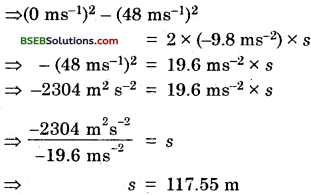By first law of motion,
V = u + gt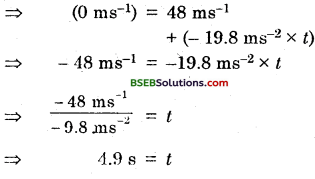⇒ 4.9 s = t
The maximum height to which the ball rises is 117.55 m.
The total time it takes to return to the surface of the Earth is .
4.9 s + 4.9 s = 9.8 s.Question 14.
A stone is released from the top of a tower of height 19.6 m. Calculate its final velocity.
Initial velocity, u = 0 ms-1
Final velocity, V = ?
Height of tower, s = 19.6 m
Acceleration due to gravity,
g = 9.8 ms2
By third law of motion,
v2 – u2 = 2 gs .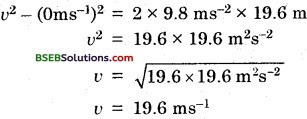v = 19.6 ms-1

Question 15.
A stone is thrown vertically upward with an initial velocity of 40 m/s. Taking g = 10 m/s2, find the maximum height reached by the stone. What is the net displacement and the total distance covered by the stone ?
(i) Initial velocity, u- 40 ms-1
Final velocity, V = 0 ms-1
Acceleration due to gravity,
g = 10 ms-2
Height readied by the stone, h = ?

By third equation of motion,
v2 – a2 = 2gh
⇒ (0 ms-1)2 – (40 ms-1)2 = 2 x (- 10 ms-2) x h
⇒ 1600 m2s-2 = – 20 ms-2x h(ii) Net displacement = 0 (Since the stone reaches back on the ground)
(iii) Total distance covered by stone = 80 m + 80 m = 160 mQuestion 16.
Calculate the force of gravitation between ” the earth and the Sun, given that the mass of the earth = 6 x 1024 kg and the sun = 2 x 1030 kg. The average distance between the two is 1.5 x 1011 m.
Mass of the earth,
m1 = 6 x 1024 kg
Mass of the sun,
m2 = 2 x 1030 kg
Distance between earth and sun, r = 1.5 x 1011 m
Gravitational constant,
G = 6.67 x 1030 Nm2 kg-2
By universal law of gravitation,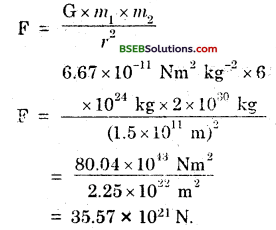Question 17.
A stone is allowed to fall from the top of a tower 100 m high and at the same time another stone is projected vertically upwards from the ground with a velocity of 25 m/s. Calculate when and yvhere the two stones meet ?
Let the two stones meet after a time t seconds at a distance of x from the ground.
Then distance covered by the stone allowed to fall from the top of the towerAnd the distance covered by the stone thrown from the ground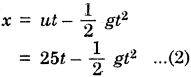Adding (1) and (2), we get
100 = 251 ⇒ t = 4 seconds.
And x = 25 x 4 – $$\frac {1}{2}$$ x 9.8 x 42 = 100 – 78.4 = 21.6 m.

Question 18.
A ball thrown up vertically returns to the thrower after 6 s. Find :
(a) the velocity with which it was thrown up.
(b) the maximum height it reaches, and
(c) its position after 4 s.
(i) Acceleration due to gravity, g = – 9.8 ms2
Time, t = 3 s (since the time to go up and return is 8 s. The time to go up = 3 s)
Final velocity, V = 0 ms-1
Initial velocity, u = ?
By first equation of motion,
V = u + gt
0 ms-1 = u + (- 9.8 ms-2) x 3 s
⇒ 0 ms-1 = u – 29.4 ms-1
⇒ u = 29.4 ms-1
Thus, the velocity with which it was thrown up = 29.4 ms-1.
Distance, s = Height, h = ?
(ii) By second equation of motion,
s = ut + $$\frac {1}{2}$$ gt2
s = 29.4 ms-1 x 3 S + $$\frac {1}{2}$$ x (-9.8 ms-2) x (3 s)2
s = 88.2 m – 44.1m
s = 44.1 m(iii) Time, t = 4 s
Distance, s = ?
By second equation of motion,
s = ut + $$\frac {1}{2}$$ gt2
s = 29.4 ms-1 x 4 s + $$\frac {1}{2}$$ x (- 9.8 ms-2) x (4 s)2
s = 117.6 m- 78.4 ni s = 39.2 m

Question 19.
In what direction does the buoyant force on an immersed in a liquid act ?
The buoyat force on an object acts in an upward direction.

Question 20.
Why does a block of plastic released under water come up to the surface of water ?
The weight of an object is the force due to gravitational attraction of the earth. When the block of plastic piece is immersed, the upward force exerted by the water on the plastic block is greater than its weight. Therefore it comes to the surface of water

Question 21.
The volume of 50 g of a sub-stance is 20 cm3. If the density of water is 1 g cm-3, will the or sink ?
Mass = 50 g
Volume = 20 cm3
Density of a substance
Density = ?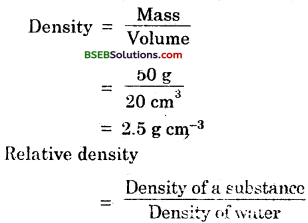Since the relative density is greater than 1, t.’ierefore the substance will sink.

Question 22.
The volume of a 500 g sealed packet is 350 cm3. Will the packet float or sink in water if the density of water is 1 g cm-3 ? What will be the rnass of the water displaced by this packet ?
(i) Mass 500 g
Volume = 350 cm3
Density = ?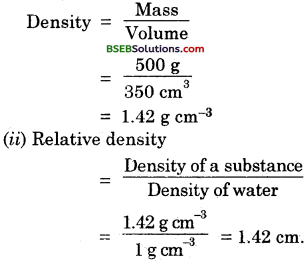(iii) Since the relative density is greater than 1, therefore the packet will sink.
(iv) The solid will displace water equal to its own volume (i.e., 350 cm3). The weight of 350 cm3 of water is 350 gram-weight. Thus, the weight of water displaced is 350 gram-weight.### Bihar Board Class 9 Science Chapter 10 Gravitation Textbook Activities

Activity 10.1

Take a piece of thread. Tie a small stone at one end. Hold the other end of the thread and whirl it round as shown in Fig. 10.2. Note the motion of the stone. Release the thread. Again note the direction of motion of the stone.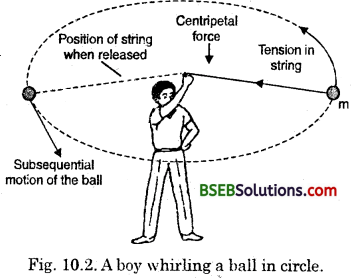Before releasing the thread, the stone moves in a circular path with a constant speed and change in direction at every point. The change in direction involves change in velocity or acceleration. The force that attracts this acceleration and keeps the body moving along the circular path, acta towards the centre. This force is Called centripetal force. When the thread is released i.e., in the absence of centripetal force, the stone flies off along the straight line. This straight line will be tangent to the circle.

Activity 10.2

Question 1.
Take a stone. Throw it upwards. It reaches a certain height and then it starts falling down.
When a stone is thrown upwards it reaches a certain height and then it starts falling down. This is due to the gravitational force.

Activity 10.3

Question 2.
Take a sheet of paper and a stone. Drop them simultaneously from the first floor of a building.
(i) What will you see? Give reason for your observation.
(ii) What will happen if the experiment is performed in a glass jar from which air has been sucked out?
(i) We will see that the paper will reach the ground a little later than the stone. This happens because the fall is through air. The air offers resistance to the motion, due to friction to the falling objects. The resistance offered to the paper is more than the resistance offered by air to the stone. Also, wind may deflect the paper side ways.
(ii) If the experiment is performed in a glass jar from which air has been sucked out, then the paper and the stone would fall at the same rate.Based on Activity 10.4

Question 1.
Take an empty plastic bottle. Close the mouth of the bottle with an air tight stopper.
(i) Push the bottle can in a bucket filled with water. What will you see ?
(ii) Push the bottle into the water. What do you feel ?
(iii) What happens when you. try to push the bottle further and further ? What does this indicate about water ?
(i) We will see that the plastic bottle will float on water.
(ii) We will feel an upward push when we push the bottle into the water.
(iii) When we try to push the bottle further and further we will find it difficult to push deeper and deeper.
This indicates that water exerts a force on the bottle in the upward direction. The upward force exerted by the water, gobs on increasing and the bottle is pushed deeper and deeper.

Question 2.
Take the empty bottle used in the above question and try to push it in a bucket filled with water so that the bottle is completely immersed.
(i) What happens when the bottle is released ?
(ii) Does the force due to gravitational attraction of the earth act on this bottle ?
(iii) If so, why the bottle does not stay immersed in water after releasing it ?
(iv) How can you have the bottle immersed in water ?
(i) When the bottle is released it bounces back.
(ii) The force due to gravitational attraction of the earth acts on the buttle in downward direction. So the
bottle is pulled downwards. But the water exerts an upward force on the bottle. Thus, the bottle is pushed upwards.
(iii) Weight of an object is the force due to gravitational attraction of the earth. When the bottle is immersed, the upward force exerted by the water on the bottle is greater than its weight. Therefore it rises up when it is released. ,
(iv) To keep the bottle completely immersed, the upward force on the bottle due to water must be balanced. This bottle be done by an externally applied force acting downwards. This force must be equal to the difference between the upward force and the weight of 5 the bottle.Activity 10.5

Question 1.
Take a beaker filled with water. Take an iron nail and place it on the surface of the water. Observe what happens.
The iron nail will sink. This means that the force due to gravitational attraction of earth on the iron nail pulls it downwards. There is upthrust of water on the nail, which pushes it upwards. But downward force acting oil the nail is greater than the upthrust of water on the nail. So, it sinks.

Activity 10.6

Question 1.
Take a beaker filled with water. Take a piece of cork and an iron nail of equal mass. Place them on the surface of water. Observe what happens ?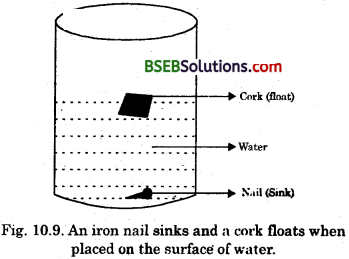The cork will float while the nail will sink. This happens because of difference in their densities. (The density of a substance is defined as the mass per unit volume.) The density of cork is less than the density of water. This means that the upthrust of water on the cork is greater than its weight. So, it floats. The density of iron nail is more than the density of water. This means that the upthrust of water in the iron nail is lesser than its weight. So, it sinks.

Activity 10.7

Question 1.
Take a piece of stone and tie it to one end of a rubber string or a spring balance. Suspend the stone by holding the balance or the string as shown in the figure 10.10 (a). Note the elongation of the string or the reading on the spring balance due to the weight of the stone. Now, slowly dip the stone in the water in a container as shown is Pig. 10.10(6). Observed what happens to elongation of the string or the reading on the balance.
We will find that the elongation of the string or the reading of the balance decreases as the stone is gradually lowered in water. However, no further change is observed once the stone gets fully immersed in the water.### Bihar Board Class 9 Science Chapter 10 Gravitation Additional Important Questions and Answers

Question 1.
Name the scientist who discovered that force is the cause of motion.
Galileo Galilei discovered that force is the cause of motion.

Question 2.
Name the physical quantity that is needed to change the speed or the direction of motion of an object.
Force is needed to change the speed or the direction of motion of an object.

Question 3.
Name the force which is responsible for the* planets to go round the sun.
Gravitational force.Question 1.
It is said that when Newton was sitting under a tree, an apple fell on him. The fall of the apple made Newton to think.
(i) What did he think?
(ii) What did he realize?
(i) He thought that if the earth can attract an apple, can it not attract the moon.
(ii) He realized that the same type of force is responsible for the attraction of an apple and the attraction of moon by the earth.

Question 2.
List the various types of forces that exist in nature.

• Force of gravity due to earth.
• Force of gravitational attraction between any two bodies possessing mass
• Electric force
• Magnetic force
• Force of friction.

Question 1.
Suppose you and your friend have mass 50 kg each. Suppose also that you are standing such that your centres of gravity are 1 m apart. Calculate the force of gravitation between you and your friend. Calculate also the force of gravity acting on you. [Mass of the earth = 6 x 1024kg, Radius of the earth = 6.4 x 106 m]
(Use F = mg = Gm Me/Re2)
Mass of you and your friend = 50 kg
each Distance between you and your friend = 1 in

Then,
Force of gravitation =Force of gravity acting on,a body.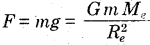Me is the mass of the earth
Re is the radius of the earth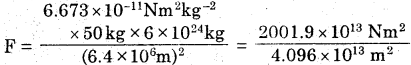= 488.74 N.